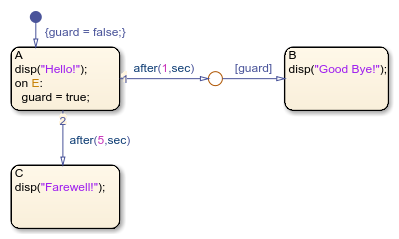## 使用时序逻辑控制图的执行

• 基于事件的时序逻辑会跟踪重复发生的事件。可以将任何显式或隐式事件用作基础事件。

• 绝对时间时序逻辑会跟踪从状态激活以来经过的时间。绝对时间时序逻辑运算符的计时取决于 Stateflow® 图的类型：

• MATLAB® 中的独立图根据挂钟时间定义绝对时间时序逻辑，其精度限制在 1 毫秒。

### 时序逻辑运算符

• 状态的 `on` 动作

• 源自某一状态的转移路径上的动作

`after`

`after(n,E)`

`n` 是正整数或计算结果为正整数值的表达式。

`E` 是运算符的基础事件。

``on after(3,E): disp("ON");``

`after(5,E)`

`after(n,tick)`

`n` 是正整数或计算结果为正整数值的表达式。

`after(7,tick)[temp > 98.6]`

`after(n,sec)`

`after(n,msec)`

`after(n,usec)`

`n` 是正实数或计算结果为正实数的表达式。

``on after(12.3,sec): temp = LOW;``
`at`

`at(n,E)`

`n` 是正整数或计算结果为正整数值的表达式。

`E` 是运算符的基础事件。

``on at(3,E): disp("ON");``

`at(5,E)`

`at(n,tick)`

`n` 是正整数或计算结果为正整数值的表达式。

`at(7,tick)[temp > 98.6]`

`at(n,sec)`

`n` 是正实数或计算结果为正实数的表达式。

``on at(12.3,sec): temp = HIGH;``
`before`

`before(n,E)`

`n` 是正整数或计算结果为正整数值的表达式。

`E` 是运算符的基础事件。

``on before(3,E): disp("ON");``

`before(5,E)`

`before(n,tick)`

`n` 是正整数或计算结果为正整数值的表达式。

`before(7,tick)[temp > 98.6]`

`before(n,sec)`

`before(n,msec)`

`before(n,usec)`

`n` 是正实数或计算结果为正实数的表达式。

``on before(12.3,sec): temp = MED;``
`every`

`every(n,E)`

`n` 是正整数或计算结果为正整数值的表达式。

`E` 是运算符的基础事件。

``on every(3,E): disp("ON");``

`every(5,E)`

`every(n,tick)`

`n` 是正整数或计算结果为正整数值的表达式。

`every(7,tick)[temp > 98.6]`

`every(n,sec)`

`n` 是正实数或计算结果为正实数的表达式。

``on every(12.3,sec): temp = temp+5;``
`temporalCount`

`temporalCount(E)`

`E` 是运算符的基础事件。

``on E: y = M(temporalCount(E));``
`temporalCount(tick)`

```en,du: M(temporalCount(tick)+1) = u;```

`temporalCount(sec)`

`temporalCount(msec)`

`temporalCount(usec)`

```en,du: y = temporalCount(msec);```
`elapsed`

`elapsed(sec)`

```en,du: y = elapsed(sec);```
`et`执行 `elapsed(sec)` 的另一种方式。

`E{disp(et);}`
`count`

`count(C)`

`C` 是计算结果为 `true``false` 的表达式。

`[count(x>=2) > 5]`

```en,du: y = count(x>5);```
`duration`

`duration(C)`

`duration(C,sec)`

`duration(C,msec)`

`duration(C,usec)`

• `C` 是计算结果为 `true``false` 的表达式。

MATLAB 中的独立图不支持时序逻辑运算符 `duration`

`[duration(x>=0) > 0.1]`

```en,du: y = duration(x>5,msec);```

### 时序逻辑示例

#### 定义时滞1. 当图唤醒时，状态 `Input` 先激活。

2. 仿真执行 5.33 毫秒后，会发生从 `Input``Output` 的转移。

3. 状态 `Input` 变为非激活，状态 `Output` 被激活。

4. 仿真执行 10.5 秒后，会发生从 `Output``Input` 的转移。

5. 状态 `Output` 变为非激活，状态 `Input` 被激活。

#### 检测已用时间• 如果输入在 t = 2 秒之前等于 1，则发生从 `Start``Fast` 的转移。

• 如果输入在 t = 2 秒和 t = 5 秒之间等于 1，则发生从 `Start``Medium` 的转移。

• 如果输入在 t = 5 秒后等于 1，则发生从 `Start``Slow` 的转移。

#### 在使能子系统中使用绝对时间时序逻辑• 信号在 t = 0 时激活子系统。

• 信号在 t = 2 时禁用子系统。

• 信号在 t = 6 时重新激活子系统。### 转移中基于事件的时序逻辑的表示法

• 触发器表示法定义仅依赖时序逻辑运算符的基础事件的转移。触发器表示法遵循以下语法：

`temporalLogicOperator(n,E)[C]`
其中：

• `temporalLogicOperator` 是一个布尔时序逻辑运算符。

• `n` 是运算符的出现次数。

• `E` 是运算符的基础事件。

• `C` 是一个可选条件表达式。

当您使用触发器表示法时，仅当图处理基础事件 `E` 的广播时才会发生转移。

• 条件表示法定义依赖基础事件和非基础事件的转移。条件表示法遵循以下语法：

`F[temporalLogicOperator(n,E) && C]`
其中：

• `temporalLogicOperator` 是一个布尔时序逻辑运算符。

• `n` 是运算符的出现次数。

• `E` 是运算符的基础事件。

• `F` 是可选的非基础事件。

• `C` 是一个可选条件表达式。

当您对非基础事件 `F` 使用条件表示法时，仅当图处理 `F` 的广播时才会发生转移。如果忽略非基础事件，则当图处理任何显式或隐式事件时都可以发生转移。

MATLAB 中的独立图不支持时序逻辑运算符的条件表示法。

`after(5,E)`

`[after(5,E)]`

### 时序逻辑的最佳做法

#### 不要在没有源状态的转移路径中使用时序逻辑

• 状态的 `on` 动作

• 源自某一状态的转移路径上的动作

#### 不要在 Simulink 模型的图中用 `at` 来表示绝对时间时序逻辑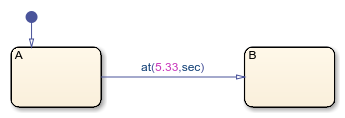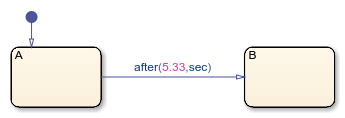#### 大参数值的意外结果

• 图具有周期性的离散采样时间。

• 图逻辑使状态保持激活状态超过 `2147418` 个时间单位。时间单位是该状态使用的任何时序逻辑表达式中的最小时间单位。例如，如果状态有两个出向转移，其中一个使用 `after(x,sec)`，另一个使用 `after(x,msec)`，则时间单位为 `msec (milliseconds)`

#### 不要在 Simulink 模型的图中用 `every` 来表示绝对时间时序逻辑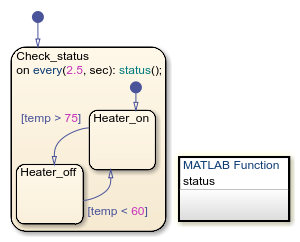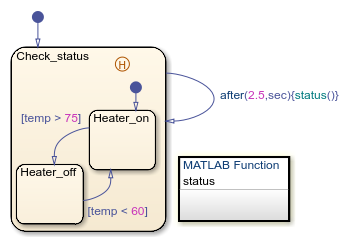#### 在 MATLAB 的独立图中，不要在具有多个源的转移路径中使用时序逻辑

MATLAB 的独立图不支持在具有多个源状态的转移路径中使用时序逻辑运算符。例如，以下独立图会产生运行时错误，因为时序逻辑表达式 `after(10,sec)` 会触发具有多个源状态的转移路径。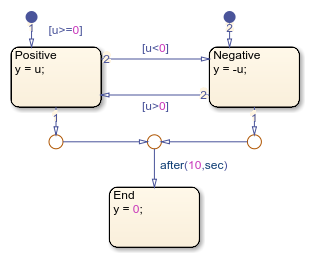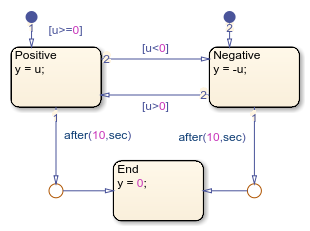#### 避免在 MATLAB 的独立图的转移路径中混合使用绝对时间时序逻辑和条件

• 如果当 `timer` 唤醒图时转移路径上的条件为 false，则图执行激活状态的 `during``on` 动作。

• 图不会重置与运算符 `after``at` 关联的 `timer` 对象。即使转移路径上的条件随后变为 true，如果没有另一个显式或隐式事件来唤醒图，转移也不会发生。